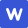2022年高考真题 数学 (新高考I卷)

|

# 数学 热门试卷

X 查看更多试卷

1、单选题
1
2
3
4
5
6
7
8
2、多选题
9
10
11
12
3、填空题
13
14
15
16
4、简答题
17
18
19
20
21
22
• 真题试卷
• 模拟试卷

1

|

A

B

C

D

D
1

|

，则（    ）

A

B

C1

D2

D
1

|

A

B

C

D

B
1

|

A

B

C

D

C
1

|

A

B

C

D

D
1

|

A1

B

C

D3

A
1

|

，则（    ）

A

B

C

D

C
1

|

A

B

C

D

C

1

|

A直线所成的角为

B直线所成的角为

C直线与平面所成的角为

D直线与平面ABCD所成的角为

A,B,D
1

|

A有两个极值点

B有三个零点

C是曲线的对称中心

D直线是曲线的切线

A,C
1

|

AC的准线为

B直线ABC相切

C

D

B,C,D
1

|

A

B

C

D

B,C

1

|

-28

1

|

### 正确答案

x=-1或y=或y=（答对其中之一即可）

1

|

ａ＞0或ａ＜-4

1

|

13

1

|

（1）求的通项公式；

（2）证明：

### 正确答案

(1)==1,=1,是公差为的等差数列，=1+(n-1)=n+,=(n+),=,n,,相乘得,n,=,n，经验证符合，=,n

（2）==2(-),++…+=2(1-+-…+-)=2-,n,,2-,++…+

1

|

（1）若，求B

（2）求的最小值．

### 正确答案

(1)===,cosAcosB-sinAsinB=sinB,cos(A+B)=sinB=-cosC=-cos=,0,B=

(2)sinB=cos(A+B)=-cosC=sin(C-),C钝角,B=C-,sinB=sin(C-)=-cosC,sinA=sin(B+C)=sin(2C-)=cos2C,由余弦定理，+=+2abcosC,=1+=1+=1+2(2+-3)1+2(2-3)=4-5

1

|

（1）求A到平面的距离；

（2）设D的中点，，平面平面，求二面角的正弦值．

### 正确答案

(1)=3=4,=,==,设A到平面的距离为h，则h===

（2）设D为,,,AB,BCAB,在RT中，∠ABC=90°，链接B，过A作AHB,则AH，BC，BCB,A=AB,AH=,A=AB=2,B=2,=2=B*BC.BC=2,以点B为原点，BC,BA,B分别为X,Y,Z建立空间直角坐标系，C(2,2,0)A(0,2,0)(0,2,2)B(1.1.1),设,(X,Y,Z),(,,),,Y=0,X+Y+Z=0,(1,0,-1),,=(0,1,-1),|cos(*)|==,sin(*)=,二面角的正弦值为

1

|

1

|

（1）求l的斜率；

（2）若，求的面积．

### 正确答案

（1）将点A代入双曲线方程得-=1，化简得-4+4=0得=2，故双曲线方程为-由题显然直线/的斜率存在，设l:y=kx+m，设P()Q()，则联立直线与双曲线得:(2-1)+4kmx+2㎡+2=0,

+=,=,+=+=+=0,化简得，2k+（m-1-2k）（+）-4（m-1）=0，故+（m-1-2k）-4（m-1）=0,即(k+1)(m+2k-1)=0,二直线l不过点A，故k=-1

（2）设直线AP的倾斜角为α，由tan∠PAQ=2，得tan=

tan∠PAQ=2，得sin∠PAQ=

=|AP||AQ|sin∠PAQ=

1

|

（1）求a

（2）证明：存在直线，其与两条曲线共有三个不同的交点，并且从左到右的三个交点的横坐标成等差数列．

### 正确答案

（1）-a,a-,由最小值得a,x(0,),递减，反之递增，x(0,),递减，反之递增，

min==a-a,min==1+,1+=a-a,h(a)=a-a-,h(a)’=1--1-=,x(0,)，h(a)递增，反之递减，h(a)min=-1,h(1)=1,a=1

(2)-a,a-,b=-x,只有一个交点，x-=b,有两个交点，x-=b，=,=,x=,,有2个相等，==-=-=-+=2